# DVB-S2 HDL LDPC Encoder

This example shows how to implement DVB-S2 LDPC encoding using Simulink® blocks that are optimized for HDL code generation.

The DVB-S2 LDPC Encoder block in this example works in conjunction with the DVB-S2 LDPC Decoder block. The output results of this example are compared with those of the ldpcEncode helper function in Satellite Communications Toolbox.

### Introduction

Digital Video Broadcast Satellite Second Generation (DVB-S2) is a European Telecommunications Standards Institute (ETSI) standard of the second generation for digital data transmission through satellites [ 1 ]. The DVB-S2 standard is designed for broadcast services, interactive services, digital satellite news gathering, and professional services. In 2005, DVB-S2 became the first standard to adopt low-density parity-check (LDPC) codes. DVB-S2 offers a powerful forward error correction (FEC) based on LDPC codes concatenated with Bose Chaudhuri Hocquenghem (BCH) codes. This mechanism allows quasi error-free operation at about 0.7 dB to 1 dB from the Shannon limit [ 1 ], which yields better decoding performance.

FEC performs outer coding with BCH codes and inner coding with LDPC codes. It accepts BBFRAMEs as inputs and outputs FECFRAMEs. A BBFRAME consists of a BBHEADER followed by a DATA FIELD. FEC coding processes each BBFRAME of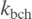bits to generate a FECFRAME of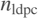bits as shown in section 5.3 [ 1 ]. The following figure shows the frame format of FECFRAME data.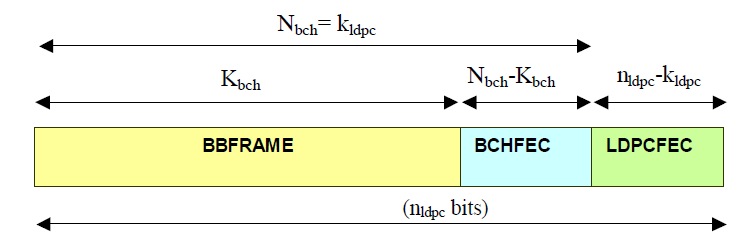The LDPC codes in the DVB-S2 standard have two block lengths. Normal frames have a block length equal to 64,800 and short frames have block length equal to 16,200. The standard specifies 11 code rates for normal frame and 10 code rates for short frame. LDPC code parameters for coded () and uncoded (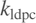) block lengths for different frames are defined in table 5a in section 5.3 of ETSI EN 302 307 [ 1 ].

### Model Architecture

This figure shows the high-level architecture block diagram of the implementation of the DVB-S2 LDPC Encoder block.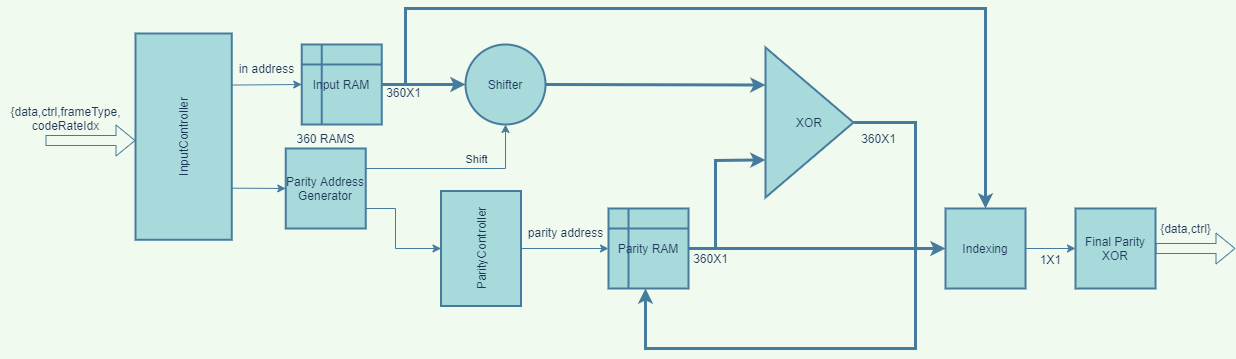This figure shows the top-level structure of the dvbs2hdlLDPCEncoder model. You can generate the HDL code for the DVB-S2 LDPC Encoder subsystem in the model.

modelName = 'dvbs2hdlLDPCEncoder'; open_system(modelName); set_param(modelName,'Open','on');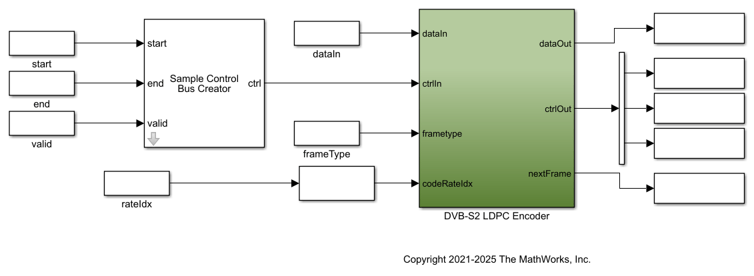DVB-S2 LDPC Encoder

The DVB-S2 LDPC Encoder subsystem accepts input data, the control signal, the frame type, and the code rate index. The frameType and codeRateIdx signals are sampled at the start of a frame. The inputController function controls the reading and writing of input data in the Input RAM subsystem and enables the encoding after writing the whole frame into the RAM. Using the parity bit addresses specified in standard Annex B, C [ 1 ], the shift values for the Circular Shifter subsystem and address for the Parity RAM subsystem are calculated and stored in the shiftLUT and ramLUT blocks respectively. Parity Address Generator subsystem generates the corresponding shift value and the address of the Parity RAM subsystem based on the input configuration of the FEC frame and code rate. ParityController function controls the parity calculation and reading of parity data. Circular Shifter subsystem shifts the data circularly, and an exclusive-OR operation is applied to the shifted data with the output of Parity RAM subsystem and stores the data in the same address. Indexing function multiplexes the input and parity bits and outputs the bits serially. The final parity bits are calculated by applying an exclusive-OR operation to the current parity bits with the previous parity bits.

set_param(modelName,'Open','off'); open_system([modelName '/DVB-S2 LDPC Encoder']);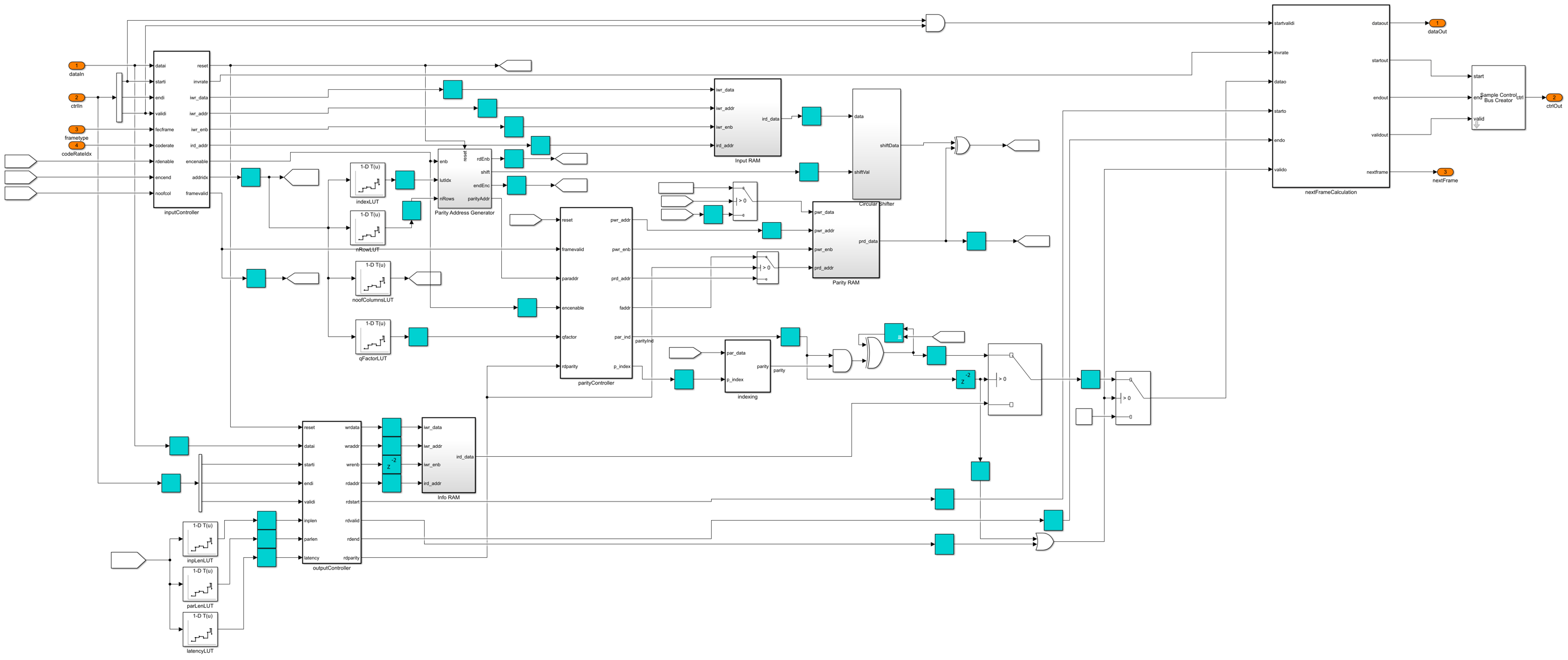The inputController function generates the encenable signal counts the number of columns and rows of parity bit addresses. The ramLUT block stores the addresses of the Parity RAM subsystem. The shiftLUT block stores the shift values of input data calculated using the following equations from the parity bit addresses specified in standard Annex B, C [ 1 ].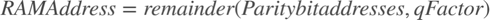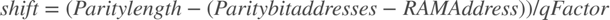set_param([modelName '/DVB-S2 LDPC Encoder'],'Open','off'); open_system([modelName '/DVB-S2 LDPC Encoder/Parity Address Generator']);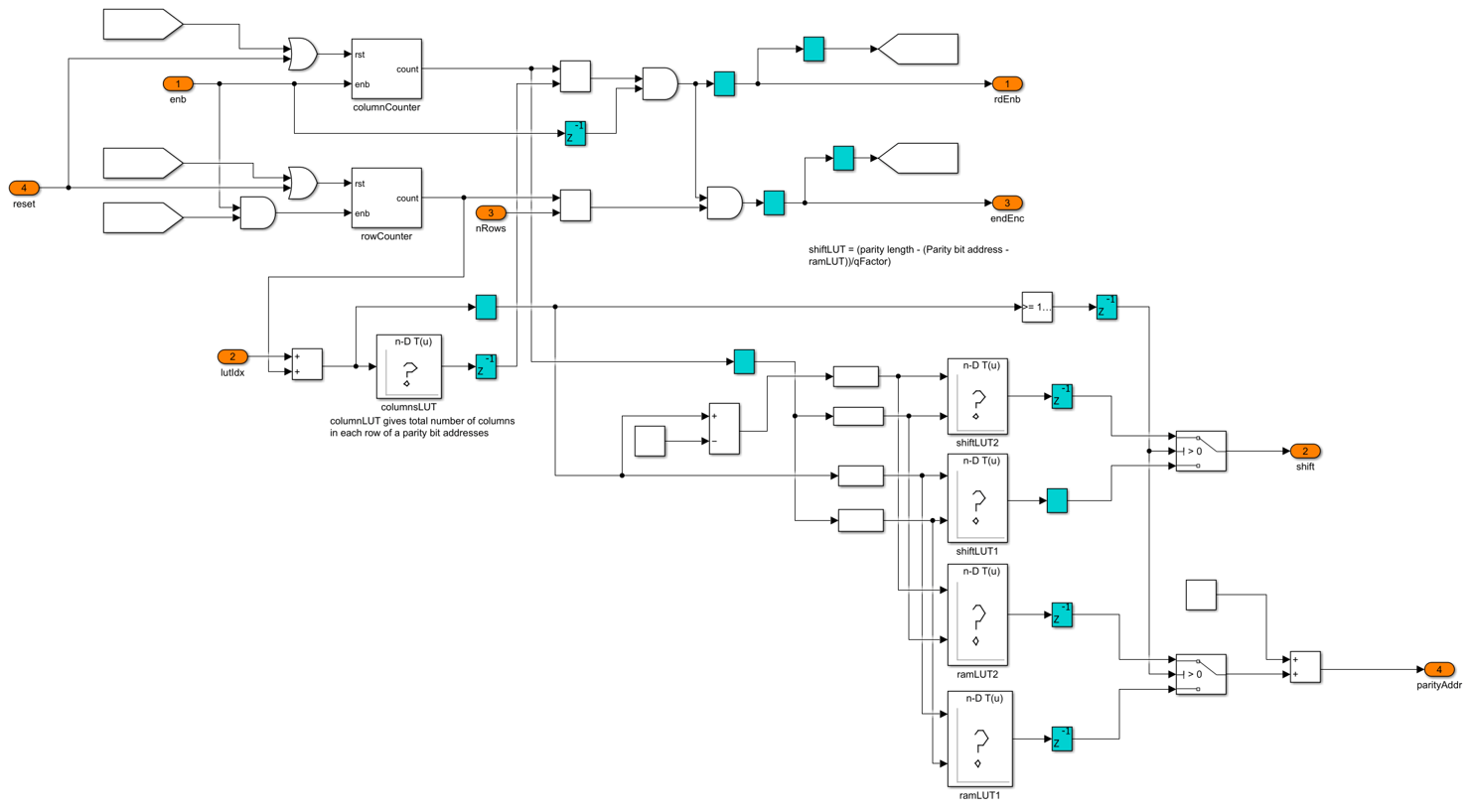Circular Shifter

Circular Shifter subsystem shifts the data based on the shift value. The circular shift network is implemented with a fixed 360 parallelism factor, which supports shift values in the range from 0 to 359. Using the selectors and multiplexers, data is shifted by powers of 2. The Circular Shifter subsystem uses each bit of shift for appropriate routing and selection of data.

set_param([modelName '/DVB-S2 LDPC Encoder/Parity Address Generator'],'Open','off'); open_system([modelName,'/DVB-S2 LDPC Encoder/Circular Shifter']);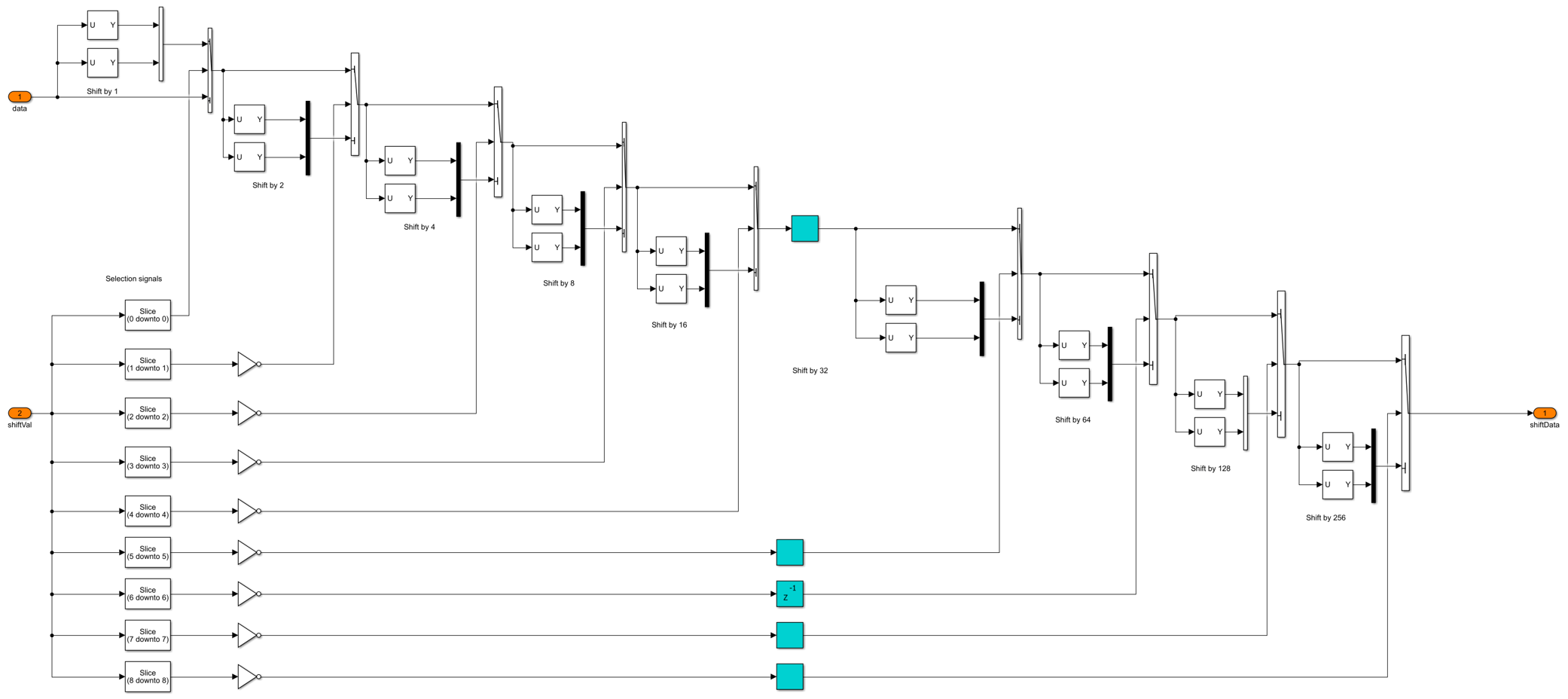### Set Up Input Variables

Choose a series of input values for the FEC frame type and a code rate according to the DVB-S2 standard. You can change the variable values in this section based on your requirements. Specify the codeRateIdx values from 0 to 10 that correspond to the codeRateSet values '1/4', '1/3', '2/5', '1/2', '3/5', '2/3', '3/4', '4/5', '5/6', '8/9', '9/10'.

fecFrameSet = {'Normal','Short'}; codeRateSet = {'1/4','1/3','2/5','1/2','3/5','2/3','3/4','4/5','5/6','8/9','9/10'}; frameType = [1 0]; % FEC frame type codeRateIdx = [1 0]; % Code rate index numFrames = 2; 

This example loads a MAT file with DVB-S2 LDPC parity matrices for the reference MATLAB® function. If the MAT file is not available on the MATLAB path, use these commands to download and unzip the MAT file.

if ~exist('dvbs2xLDPCParityMatrices.mat','file') if ~exist('s2xLDPCParityMatrices.zip','file') url = 'https://ssd.mathworks.com/supportfiles/spc/satcom/DVB/s2xLDPCParityMatrices.zip'; websave('s2xLDPCParityMatrices.zip',url); unzip('s2xLDPCParityMatrices.zip'); end addpath('s2xLDPCParityMatrices'); end 

### Generate Input Data

Generate inputs for the ldpcEncode helper function with the specified frame type and code rate variables. Create vectors of the frame type and code rate index using the frameType and codeRateIdx variables, respectively. Convert the frames of input data to samples with a control bus signal that indicates the frame boundaries. Provide these vectors and control bus as input to the DVB-S2 LDPC Encoder subsystem.

The encFrameGap variable in the script accommodates the latency of the DVB-S2 LDPC Encoder subsystem for the specified block length and code rate.

% Initialize inputs fecFrameType = fecFrameSet(frameType+1); codeRate = codeRateSet(codeRateIdx+1); msg = {numFrames}; % Input to ldpcEncode function refOut = cell(1,numFrames); % Output from ldpcEncode function encSampleIn = []; encStartIn = []; encEndIn = []; encValidIn = []; fFrameIn = []; codeRateIn = []; for ii = 1:numFrames fFrame = fecFrameType{ii}; % Input and code word length calculation if strcmpi(fFrame,'Normal') cwLen = 64800; R = str2num(codeRate{ii}); %#ok<*ST2NM> else cwLen = 16200; ReffList = [1/5 1/3 2/5 4/9 3/5 2/3 11/15 7/9 37/45 8/9]; RactList = [1/4 1/3 2/5 1/2 3/5 2/3 3/4 4/5 5/6 8/9]; Reff = ReffList(RactList == str2num(codeRate{ii})); R = Reff(1); end inpLen = cwLen*R; % Input bits generation msg{ii} = (randi([0 1],inpLen,1)); % LDPC encoding refOut{ii} = satcom.internal.dvbs.ldpcEncode(int8(msg{ii}),codeRate{ii},cwLen); % Value of 2000 is selected to accommodate the maximum latency of the % block considering different frame type and code rate configurations encFrameGap = cwLen + 2000; encSampleIn = [encSampleIn msg{ii}' zeros(1,encFrameGap)]; %#ok<*AGROW> encStartIn = logical([encStartIn 1 zeros(1,inpLen-1) zeros(1,encFrameGap)]); encEndIn = logical([encEndIn zeros(1,inpLen-1) 1 zeros(1,encFrameGap)]); encValidIn = logical([encValidIn ones(1,inpLen) zeros(1,encFrameGap)]); fFrameIn = logical([fFrameIn repmat(frameType(ii),1,inpLen) zeros(1,encFrameGap)]); codeRateIn = [codeRateIn repmat(codeRateIdx(ii),1,inpLen) zeros(1,encFrameGap)]; end dataIn = timeseries(logical(encSampleIn')); startIn = timeseries(encStartIn); endIn = timeseries(encEndIn); validIn = timeseries(encValidIn); frameTypeIn = timeseries(fFrameIn); codeRateIdxIn = timeseries(codeRateIn); [columnSum,ramLUT,shiftLUT] = columnShiftRAMLUT(1); simTime = length(encValidIn); 

The DVB-S2 LDPC Encoder subsystem contains the implementation of the DVB-S2 LDPC Encoder block. Running the model imports the input signal variables dataIn, startIn, endIn, validIn, frameTypeIn, codeRateIdxIn, and simTime to the block from the script and exports a stream of encoded output samples encOut and a control bus containing startOut, endOut, and validOut signals from the block to the MATLAB® workspace.

enc = sim(modelName); 

### Compare Simulink Block Output with MATLAB Function Output

Convert the streaming data output of the DVB-S2 LDPC Encoder subsystem to frames. Compare the frames with the output of the ldpcEncode helper function.

startIdx = find(squeeze(enc.startOut)); endIdx = find(squeeze(enc.endOut)); encData = squeeze(enc.encOut); encHDL = {numFrames}; for ii = 1:numFrames idx = startIdx(ii):endIdx(ii); encHDL{ii} = encData(idx); HDLOutput = double(encHDL{ii}(1:length(refOut{ii}))); error = sum(abs(double(refOut{ii})-HDLOutput(:))); fprintf('Encoded %s FEC frame and code rate %s: Output data differs by %d bits\n',fecFrameType{ii},codeRate{ii},error); end h = warning('off','MATLAB:rmpath:DirNotFound'); rmpath('s2xLDPCParityMatrices'); warning(h);clear h; 
Encoded Short FEC frame and code rate 1/3: Output data differs by 0 bits Encoded Normal FEC frame and code rate 1/4: Output data differs by 0 bits 

### HDL Code Generation (Generate HDL Code)

To check and generate HDL for this example, you must have an HDL Coder™ product. Use the makehdl and makehdltb commands to generate the HDL code and test bench for the DVB-S2 LDPC Encoder subsystem.

The DVB-S2 LDPC Encoder subsystem is synthesized on a Xilinx® Xilinx Zynq® UltraScale+ MPSoC ZCU102 evaluation board. The resource utilization results are shown in the table below.

 F = table(... categorical({'Slice LUT';'Slice Registers';'RAMB36';'DSP'; ... 'Max. Frequency (MHz)'}) ,... categorical({'6933';'4677';'23';'0';'468.34'}), ... 'VariableNames',{'Resources','Values'}); disp(F); 
 Resources Values ____________________ ______ Slice LUT 6933 Slice Registers 4677 RAMB36 23 DSP 0 Max. Frequency (MHz) 468.34 

Latency

The latency of the DVB-S2 LDPC Encoder model varies with FEC frame types and code rate configurations.

This figure shows the latency of the block when the input frameType is Normal and codeRateIdx is 3.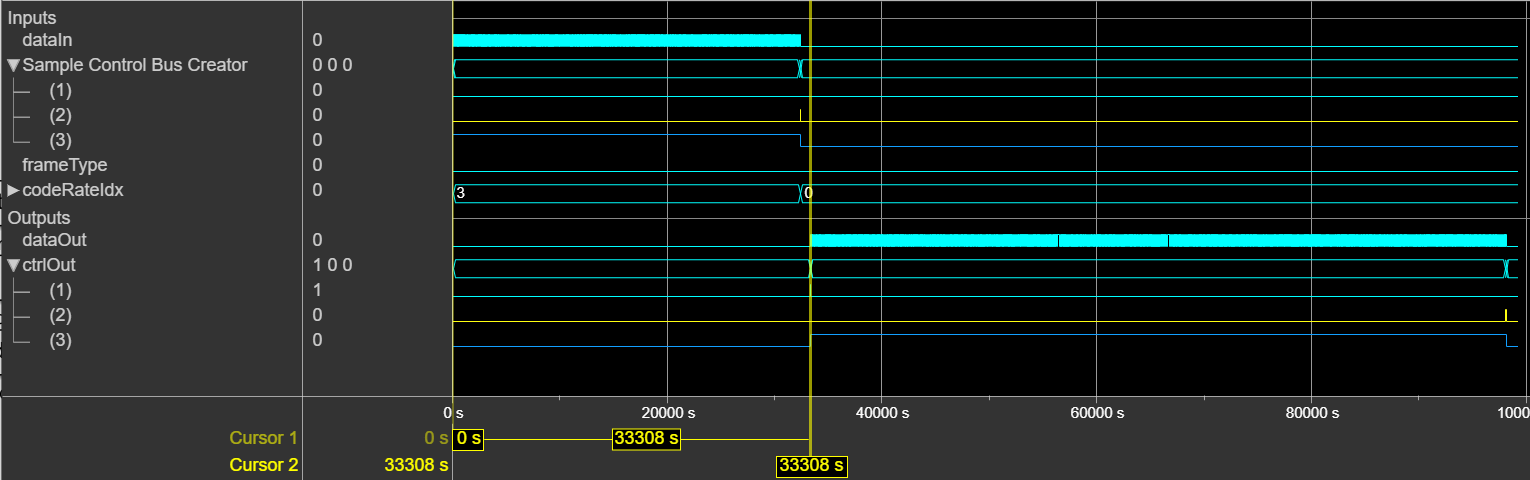The following display shows the latency of the DVB-S2 LDPC Encoder block for different FEC frame types and code rate configurations.

F = table(... categorical({'1/4';'1/3';'2/5';'1/2';'3/5';'2/3';'3/4';'4/5';'5/6';'8/9';'9/10'}), ... categorical({'16748';'22328';'26792';'33308';'40184';'44168';'49688';'53000';'55208';'58608';'59336'}), ... categorical({'3374';'5588';'6704';'7378';'10052';'11048';'12104';'12818';'13570';'14658'; '-'}), ... 'VariableNames',{'Code Rate','Normal FEC Frame','Short FEC Frame'}); disp(F); 
 Code Rate Normal FEC Frame Short FEC Frame _________ ________________ _______________ 1/4 16748 3374 1/3 22328 5588 2/5 26792 6704 1/2 33308 7378 3/5 40184 10052 2/3 44168 11048 3/4 49688 12104 4/5 53000 12818 5/6 55208 13570 8/9 58608 14658 9/10 59336 - 

### References

1. ETSI Standard EN 302 307-1 V1.4.1(2014-11): Digital Video Broadcasting (DVB); Second generation framing structure, channel coding and modulation systems for Broadcasting, Interactive Services, News Gathering and other broadband satellite applications (DVB-S2).